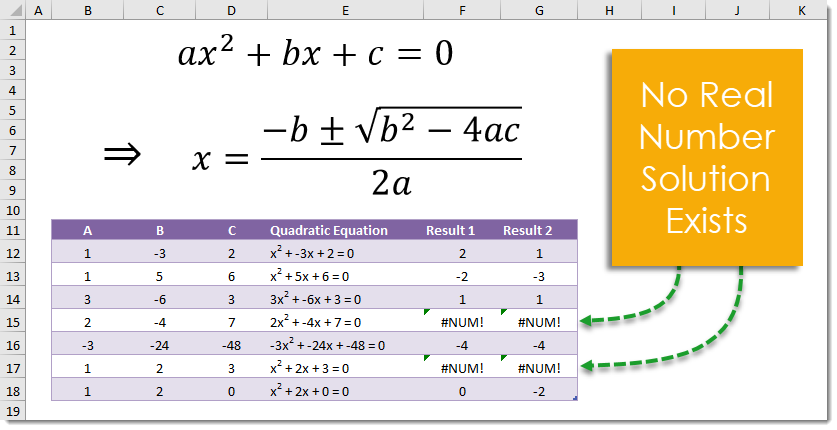## Example

``` =(-C12+SQRT(C12^2-4*B12*D12))/(2*B12) ```

## Generic Formula

There are two possible solutions for any quadratic equation. This is one possible solution.

``` =(-B+SQRT(B^2-4*A*C))/(2*A) ```

This is the other possible solution.

``` =(-B-SQRT(B^2-4*A*C))/(2*A) ```

• A – This is the coefficient of the squared term in the quadratic equation.
• B – This is the coefficient of the single powered term in the quadratic equation.
• C – This is the constant in the quadratic equation.

## What It Does

These formulas will give the solutions to a quadratic equation of the form Ax^2 + Bx + C = 0.

## How It Works

This is a simple algebraic formula and uses the SQRT function which returns the square root of a given number and the ^ operator which raises a given number to a given power.

In our example x^2 – 3x + 2 = 0 the solution is given by =(-(-3)+SQRT(-3^2-4*1*2))/(2*1) which results in 2. Similarly the other solution is 1.

Not every quadratic equation has a solution in the real numbers, when this is the case the formula will result in a #NUM! error.